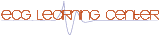Normal ECG Characteristics and Measurement Abnormalities (Lessons III and IV)

## Question 1: What is the PR interval in this ECG?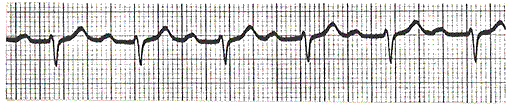A. 0.12 sec
B. 0.16 sec
C. 0.20 sec
D. 0.28 sec
E. 0.50 sec

## Question 2: What is the QRS duration seen here?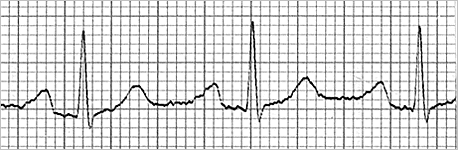A. 0.04 sec
B. 0.06 sec
C. 0.10 sec
D. 0.12 sec
E. 0.14

## Question 3: What is the QT interval of this ECG, and is it normal for this heart rate?A. 0.34 sec & no
B. 0.34 sec & yes
C. 0.40 sec & no
D. 0.40 sec & yes
E. 0.48 sec & maybe

## Question 4: What is the PR interval of this ECG?A. 0.08 sec
B. 0.12 sec
C. 0.22 sec
D. 0.28 sec
E. 80 msec

## Question 5: What is the QRS duration seen here?A. 0.04 sec
B. 0.08 sec
C. 0.12 sec
D. 0.14 sec
E. 0.16 sec

## Question 6: What is the QT Interval in this ECG strip?A. 0.34 sec
B. 0.40 sec
C. 0.44 sec
D. 0.48 sec
E. 0.20 sec

## Question 7: What is the approximate heart rate?A. 50 bpm
B. 65 bpm
C. 75 bpm
D. 90 bpm
E. 100 bpm

## Question 8: All of the following must be included in ECG interpretation except:

A. Conduction Analysis
B. PU interval
C. Waveforms
D. Rhythm Analysis
E. QT interval

## Question 9: What potentially fatal abnormality can be seen in this ECG?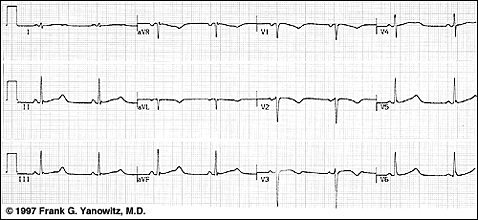A. Increased PR interval
B. Poor R wave progression (V1 - V3)
C. Increased QT interval
D. Decreased QT interval
E. Increased P wave amplitude

## Question 10: What is the approximate heart rate of this ECG?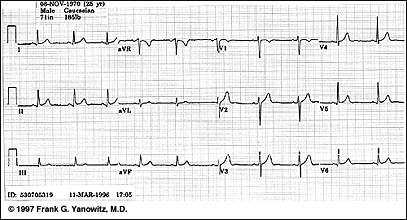A. 55 bpm
B. 70 bpm
C. 90 bpm
D. 100 bpm
E. 110 bpm